# xpath_node_set_raw Class Reference

List of all members.

## Public Member Functions

xpath_node_set_raw ()
xpath_node * begin () const
xpath_node * end () const
bool empty () const
size_t size () const
xpath_node first () const
void push_back (const xpath_node &node, xpath_allocator *alloc)
void append (const xpath_node *begin_, const xpath_node *end_, xpath_allocator *alloc)
void sort_do ()
void truncate (xpath_node *pos)
void remove_duplicates ()
xpath_node_set::type_t type () const
void set_type (xpath_node_set::type_t value)

## Detailed Description

Definition at line 6300 of file pugixml.cpp.

## Constructor & Destructor Documentation

 xpath_node_set_raw::xpath_node_set_raw ( ) ` [inline]`

Definition at line 6309 of file pugixml.cpp.

## Member Function Documentation

 void xpath_node_set_raw::append ( const xpath_node * begin_, const xpath_node * end_, xpath_allocator * alloc ) ` [inline]`

Definition at line 6352 of file pugixml.cpp.

References count, xpath_allocator::reallocate(), and size_.

Referenced by xpath_ast_node::eval_node_set().

Here is the call graph for this function: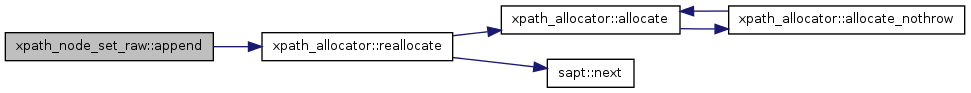Here is the caller graph for this function: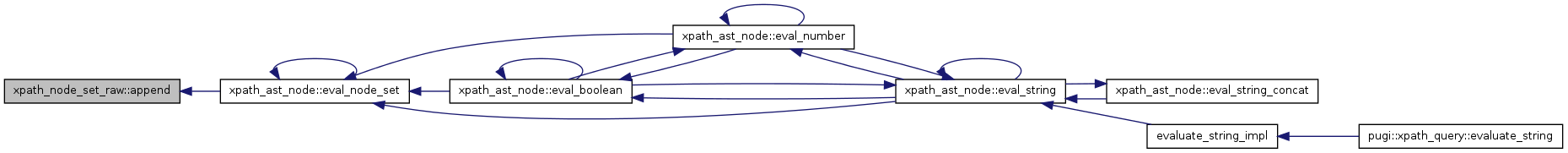xpath_node* xpath_node_set_raw::begin ( ) const` [inline]`

Definition at line 6312 of file pugixml.cpp.

Referenced by xpath_ast_node::eval_node_set(), and xpath_ast_node::eval_number().

Here is the caller graph for this function: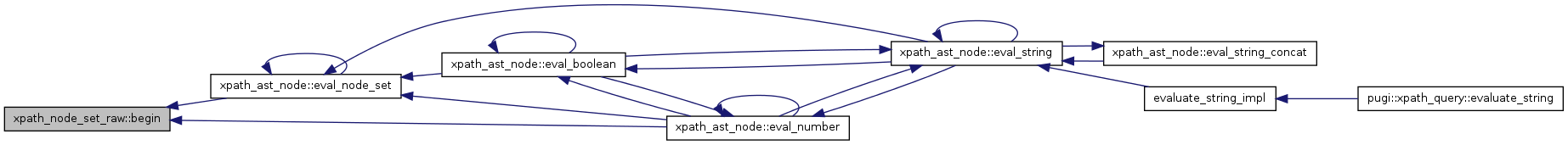bool xpath_node_set_raw::empty ( ) const` [inline]`

Definition at line 6320 of file pugixml.cpp.

Referenced by xpath_ast_node::eval_boolean(), and xpath_ast_node::eval_string().

Here is the caller graph for this function: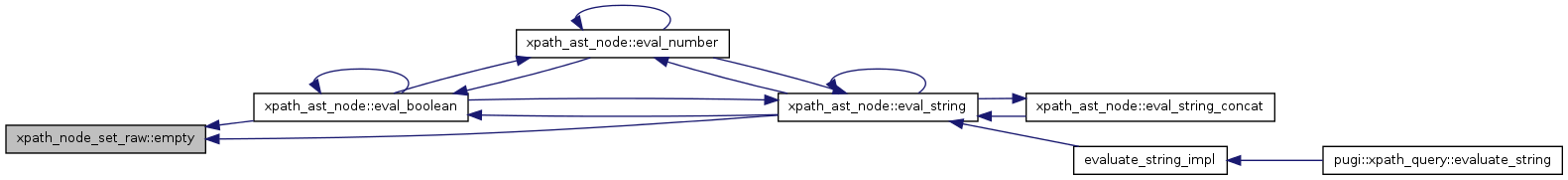xpath_node* xpath_node_set_raw::end ( ) const` [inline]`

Definition at line 6316 of file pugixml.cpp.

Referenced by xpath_ast_node::eval_node_set(), and xpath_ast_node::eval_number().

Here is the caller graph for this function: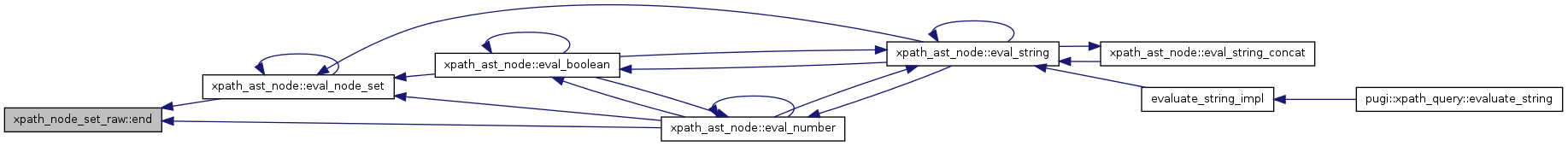xpath_node xpath_node_set_raw::first ( ) const` [inline]`

Definition at line 6328 of file pugixml.cpp.

References xpath_first().

Referenced by xpath_ast_node::eval_string().

Here is the call graph for this function: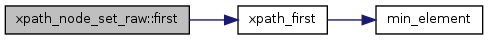Here is the caller graph for this function: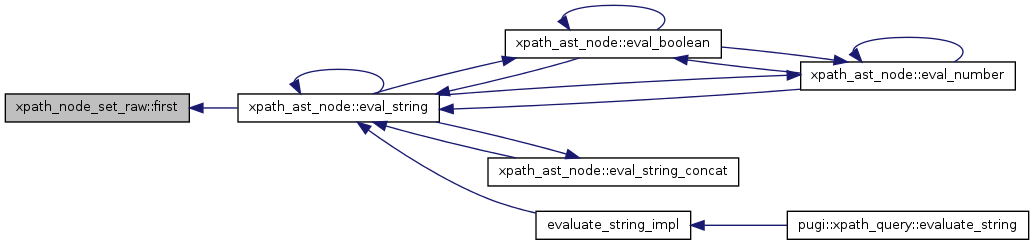void xpath_node_set_raw::push_back ( const xpath_node & node, xpath_allocator * alloc ) ` [inline]`

Definition at line 6332 of file pugixml.cpp.

References xpath_allocator::reallocate().

Referenced by xpath_ast_node::eval_node_set().

Here is the call graph for this function: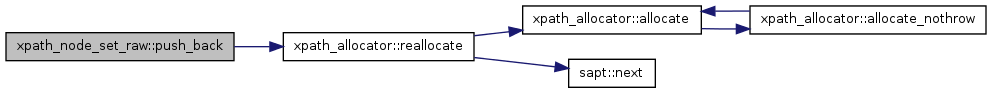Here is the caller graph for this function: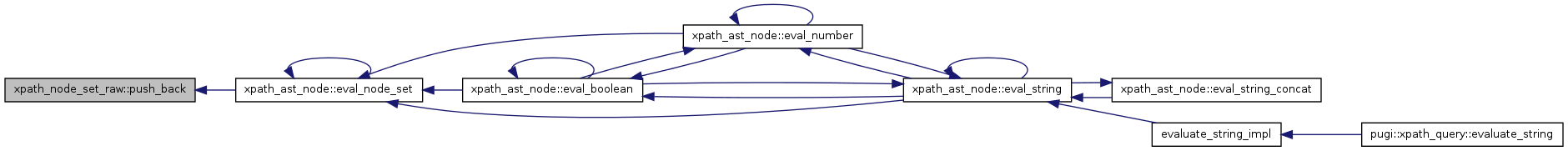void xpath_node_set_raw::remove_duplicates ( ) ` [inline]`

Definition at line 6382 of file pugixml.cpp.

References sort(), and unique().

Referenced by xpath_ast_node::eval_node_set().

Here is the call graph for this function: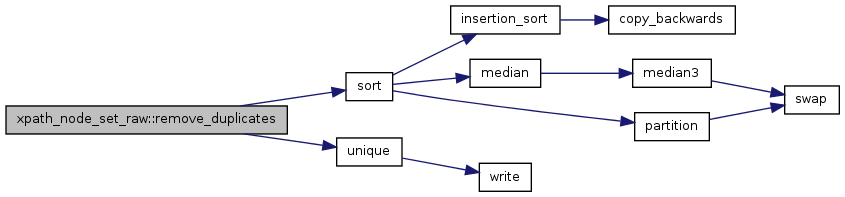Here is the caller graph for this function: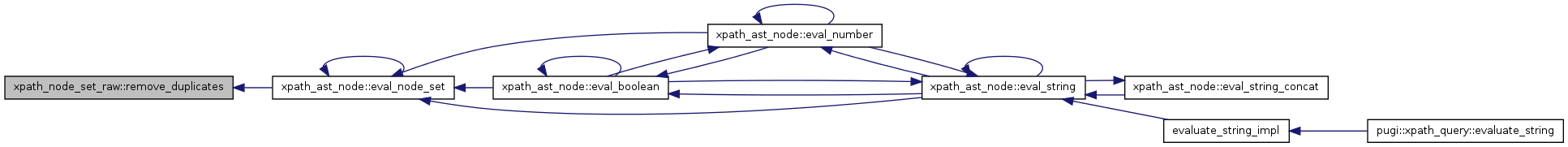void xpath_node_set_raw::set_type ( xpath_node_set::type_t value ) ` [inline]`

Definition at line 6393 of file pugixml.cpp.

Referenced by xpath_ast_node::eval_node_set().

Here is the caller graph for this function: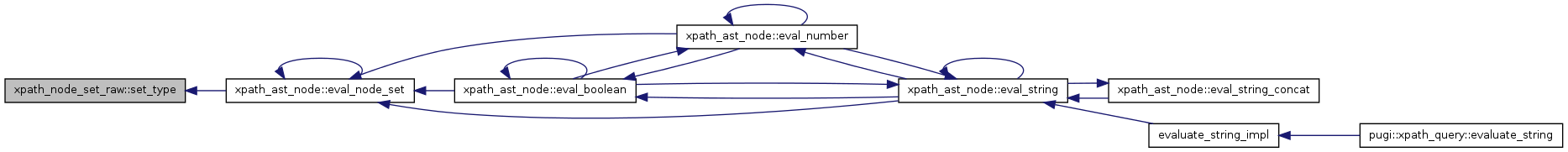size_t xpath_node_set_raw::size ( ) const` [inline]`

Definition at line 6324 of file pugixml.cpp.

Referenced by xpath_ast_node::eval_number().

Here is the caller graph for this function: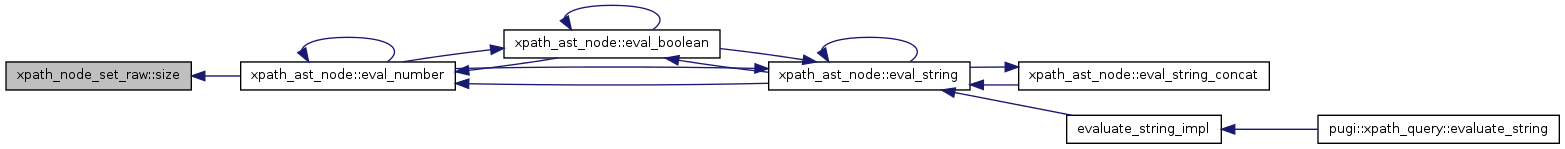void xpath_node_set_raw::sort_do ( ) ` [inline]`

Definition at line 6372 of file pugixml.cpp.

References xpath_sort().

Referenced by xpath_ast_node::eval_node_set().

Here is the call graph for this function: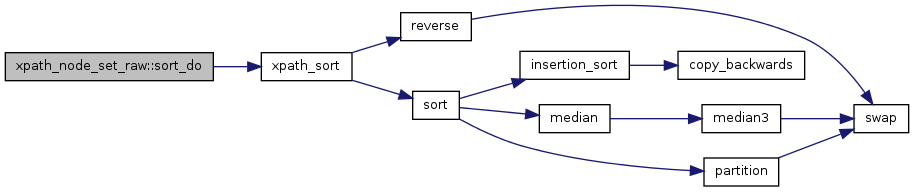Here is the caller graph for this function: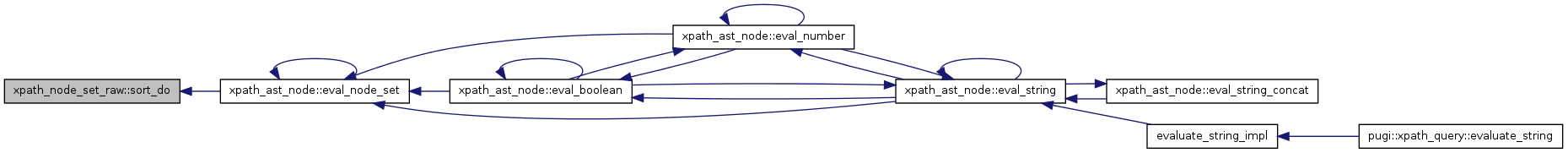void xpath_node_set_raw::truncate ( xpath_node * pos ) ` [inline]`

Definition at line 6376 of file pugixml.cpp.

 xpath_node_set::type_t xpath_node_set_raw::type ( ) const` [inline]`

Definition at line 6389 of file pugixml.cpp.

The documentation for this class was generated from the following file:
• /disk4/html/www/moses/doxygen/mosesdecoder/phrase-extract/extract-mixed-syntax/pugixml.cpp

Generated on Thu Jul 6 00:32:04 2017 for Moses by1.5.9